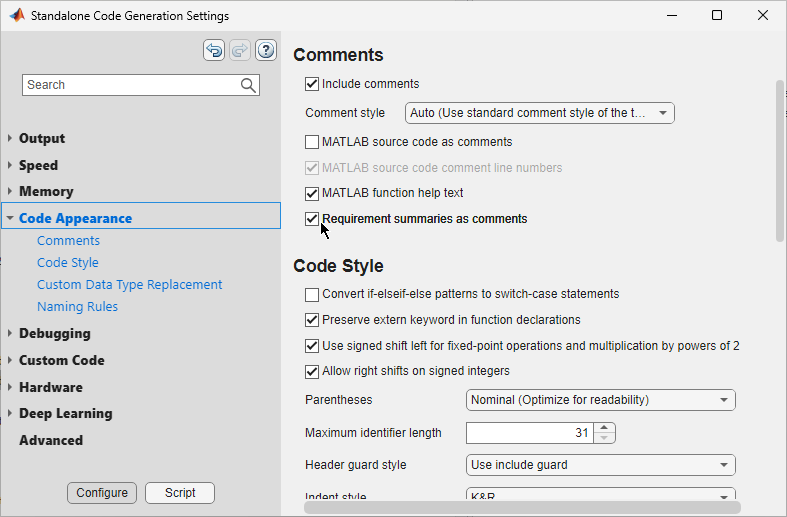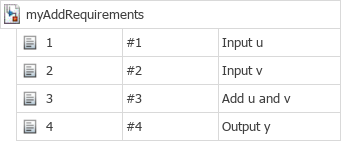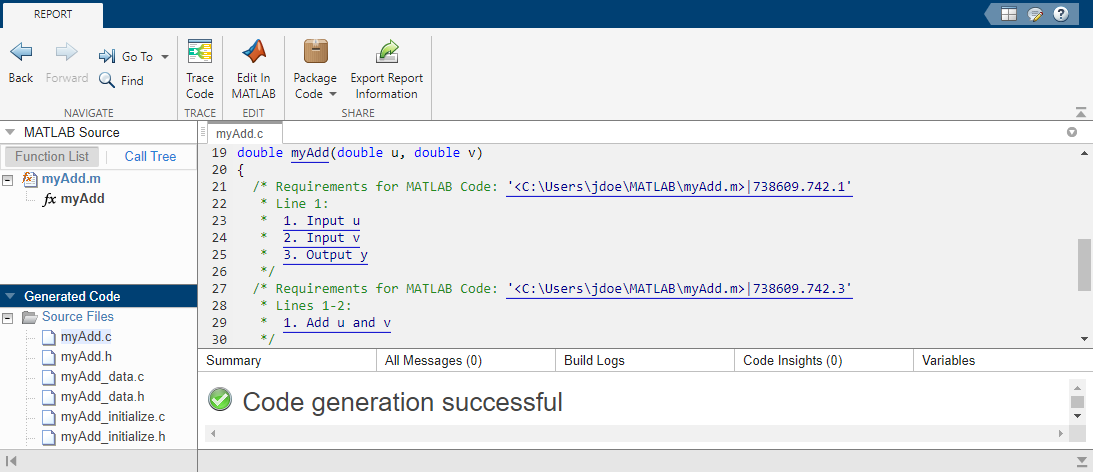## Requirements Traceability for Code Generated from MATLAB Code

When you generate C/C++ code from MATLAB® code that has links to requirements, you can include comments in the generated code that contain information about the requirements and the linked MATLAB code ranges. When you view the generated code from a code generation report, the comments are hyperlinks that you can use to navigate to the requirement and the linked MATLAB code range.

Note

To include requirement comments in generated code, you must have MATLAB Coder™ and Embedded Coder®.

### Include Requirement Comments in Generated Code

If your MATLAB code contains links to requirements, you can include requirement comments when you generate code by using the MATLAB Coder app or the `codegen` (MATLAB Coder) function. For more information, see Generate C Code by Using the MATLAB Coder App (MATLAB Coder) and Generate C Code at the Command Line (MATLAB Coder).

#### Include Requirement Comments by Using the MATLAB Coder App

To include requirement comments in generated code by using the MATLAB Coder (MATLAB Coder) app:

1. Open the MATLAB Coder app. In the Apps tab, under Code Generation, click . Alternatively, at the MATLAB command prompt, enter `coder` (MATLAB Coder).

2. In the Generate code for function field, enter the name of your MATLAB function, then click . For more information, see Open the MATLAB Coder App and Select Source Files (MATLAB Coder).

3. Define the input types for the function, then click . For more information, see Define Input Types (MATLAB Coder).

4. Check for run-time issues by clicking . If no issues are detected, click . For more information, see Check for Run-Time Issues (MATLAB Coder).

5. In the Build type menu, select one of these options:

• `Source Code`

• `Static Library (.lib)`

• `Dynamic Library (.dll)`

• `Executable (.exe)`

Note

You cannot include requirement comments in generated MEX functions.

6. To include requirement comments in the generated code, click . In the left pane, click . Under Comments, ensure that Include comments is selected, then select Requirement summaries as comments.7. To generate a code generation report, in the left pane, click . Under Code Generation Report, ensure that Always create a report is selected.

8. After you configure any additional configuration parameters, click to close the code configuration parameters menu, then click to generate the code. For more information, see Generate C Code (MATLAB Coder).

Suppose that you want to generate code and include requirement comments for a MATLAB function called `myAdd` by using the `codegen` (MATLAB Coder) function.

```function y = myAdd(u,v) %#codegen y = u + v; end```
Suppose that the function has links to these requirements:To include requirement comments in the generated code:

1. Use `coder.config` (MATLAB Coder) with the `ecoder` flag set to `true` to create a `coder.EmbeddedCodeConfig` (MATLAB Coder) object. You can use LIB, DLL, or EXE build types.

`cfg = coder.config("lib","ecoder",true);`

2. Set the `ReqsInCode (MATLAB Coder)` property of the `coder.EmbeddedCodeConfig` object to `true`.

`cfg.ReqsInCode = true;`

3. Set any additional code configuration parameters by modifying the properties of the `coder.EmbeddedCodeConfig` object. For more information, see Generate C Code at the Command Line (MATLAB Coder).

4. Define function input data types and sizes with `coder.typeof` (MATLAB Coder). For more information, see Defining Input Types (MATLAB Coder).

```utype = coder.typeof(1); vtype = coder.typeof(1);```

5. Generate the code by using `codegen` (MATLAB Coder). Use these flags as input arguments:

• `-config` to specify the code configuration object to use during code generation

• `-args` to specify the function input types and sizes

• `-launchreport` to generate and launch the code generation report

`codegen myAdd -config cfg -args {utype,vtype} -launchreport`

### View Comments in Generated Code

You can view the requirement comments by opening the generated entry-point C file, which has the same name as the MATLAB entry-point function. Each comment corresponds to a requirement link. The comment includes the:

• Full file path to the MATLAB function

• ID that represents the linked code range

• Lines of MATLAB code that are linked to the requirement

• Requirement summary

If multiple requirements are linked to the same code range, the comment lists the requirement summaries as a numbered list under the information about the linked code range.

For example, suppose you include requirement comments when you generate code from the `myAdd` function. The generated `myAdd.c` entry-point file contains comments that correspond to the requirement links:

```double myAdd(double u, double v) { /* Requirements for MATLAB Code: '<C:\Users\jdoe\MATLAB\myAdd.m>|738609.742.1' * Line 1: * 1. Input u * 2. Input v * 3. Output y */ /* Requirements for MATLAB Code: '<C:\Users\jdoe\MATLAB\myAdd.m>|738609.742.3' * Line 2: * 1. Add u and v */ return u + v; }```

### Navigate to Requirements from Code Generation Report

MATLAB Coder code generation reports allow you to view the generated C/C++ code and trace the generated code to MATLAB source code. For more information, see Code Generation Reports (MATLAB Coder).

When you view the generated entry-point C file in a MATLAB Coder code generation report, the requirement comments are hyperlinks that you can use to navigate to the linked requirements in the Requirements Editor and the linked MATLAB code range in the MATLAB Editor.Alternatively, you can trace between the generated code and the MATLAB source code without leaving the code generation report by using the button. For more information, see Interactively Trace Between MATLAB Code and Generated C/C++ Code (Embedded Coder).